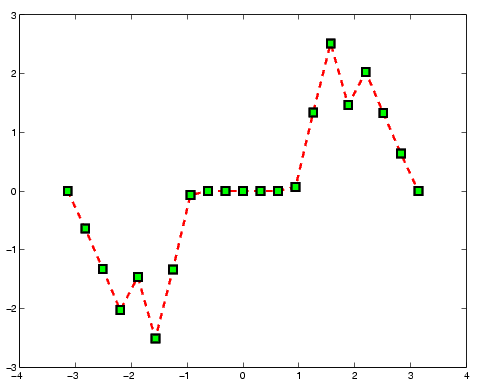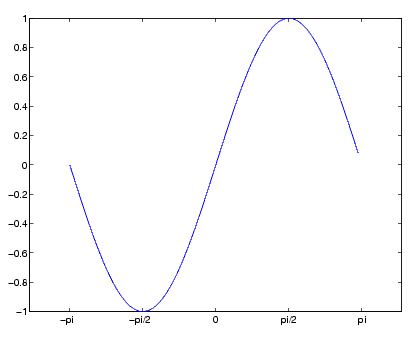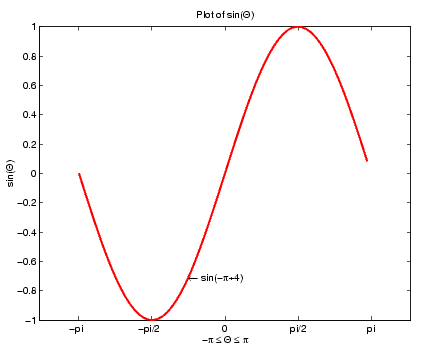MATLAB Function Referenceplot

Linear 2-D plot

Syntax

• plot(Y)
plot(X1,Y1,...)
plot(X1,Y1,LineSpec,...)
plot(...,'PropertyName',PropertyValue,...)
plot(axes_handle,...)
h = plot(...)
hlines = plot('v6',...)


Description

plot(Y)  plots the columns of Y versus their index if Y is a real number. If Y is complex, plot(Y) is equivalent to plot(real(Y),imag(Y)). In all other uses of plot, the imaginary component is ignored.

plot(X1,Y1,...)  plots all lines defined by Xn versus Yn pairs. If only Xn or Yn is a matrix, the vector is plotted versus the rows or columns of the matrix, depending on whether the vector's row or column dimension matches the matrix.

plot(X1,Y1,LineSpec,...)  plots all lines defined by the Xn,Yn,LineSpec triples, where LineSpec is a line specification that determines line type, marker symbol, and color of the plotted lines. You can mix Xn,Yn,LineSpec triples with Xn,Yn pairs: plot(X1,Y1,X2,Y2,LineSpec,X3,Y3).

 Note    See LineSpec for a list of line style, marker, and color specifiers.

plot(...,'PropertyName',PropertyValue,...)  sets properties to the specified property values for all lineseries graphics objects created by plot. (See the "Examples" section for examples.)

plot(axes_handle,...)  plots into the axes with handle axes_handle instead of the current axes (gca).

h = plot(...)  returns a column vector of handles to lineseries graphics objects, one handle per line.

Backward Compatible Version

hlines = plot('v6',...)  returns the handles to line objects instead of lineseries objects.

Remarks

If you do not specify a color when plotting more than one line, plot automatically cycles through the colors in the order specified by the current axes ColorOrder property. After cycling through all the colors defined by ColorOrder, plot then cycles through the line styles defined in the axes LineStyleOrder property.

The default LineStyleOrder property has a single entry (a solid line with no marker).

Cycling Through Line Colors and Styles

By default, MATLAB resets the ColorOrder and LineStyleOrder properties each time you call plot. If you want changes you make to these properties to persist, then you must define these changes as default values. For example,

• set(0,'DefaultAxesColorOrder',[0 0 0],...
'DefaultAxesLineStyleOrder','-|-.|--|:')


sets the default ColorOrder to use only the color black and sets the LineStyleOrder to use solid, dash-dot, dash-dash, and dotted line styles.

Prevent Resetting of Color and Styles with hold all

The all option to the hold command prevents the ColorOrder and LineStyleOrder from being reset in subsequent plot commands. In the following sequence of commands, MATLAB continues to cycle through the colors defined by the axes ColorOrder property (see above).

• plot(rand(12,2))
hold all
plot(randn(12,2))


Examples

Specifying the Color and Size of Markers

You can also specify other line characteristics using graphics properties (see line for a description of these properties):

• LineWidth -- Specifies the width (in points) of the line.
• MarkerEdgeColor -- Specifies the color of the marker or the edge color for filled markers (circle, square, diamond, pentagram, hexagram, and the four triangles).
• MarkerFaceColor -- Specifies the color of the face of filled markers.
• MarkerSize -- Specifies the size of the marker in units of points.

For example, these statements,

• x = -pi:pi/10:pi;
y = tan(sin(x)) - sin(tan(x));
plot(x,y,'--rs','LineWidth',2,...
'MarkerEdgeColor','k',...
'MarkerFaceColor','g',...
'MarkerSize',10)


produce this graph.Specifying Tick-Mark Location and Labeling

You can adjust the axis tick-mark locations and the labels appearing at each tick. For example, this plot of the sine function relabels the x-axis with more meaningful values:

• x = -pi:.1:pi;
y = sin(x);
plot(x,y)
set(gca,'XTick',-pi:pi/2:pi)
set(gca,'XTickLabel',{'-pi','-pi/2','0','pi/2','pi'})


Now add axis labels and annotate the point -pi/4, sin(-pi/4).Adding Titles, Axis Labels, and Annotations

MATLAB enables you to add axis labels and titles. For example, using the graph from the previous example, add an x- and y-axis label:

• xlabel('-\pi \leq \Theta \leq \pi')
ylabel('sin(\Theta)')
title('Plot of sin(\Theta)')
text(-pi/4,sin(-pi/4),'\leftarrow sin(-\pi\div4)',...
'HorizontalAlignment','left')


Now change the line color to red by first finding the handle of the line object created by plot and then setting its Color property. In the same statement, set the LineWidth property to 2 points.

• set(findobj(gca,'Type','line','Color',[0 0 1]),...
'Color','red',...
'LineWidth',2)axis, bar, grid, hold, legend, line, LineSpec, loglog, plot3, plotyy, semilogx, semilogy, subplot, title, xlabel, xlim, ylabel, ylim, zlabel, zlim, stem
See the text String property for a list of symbols and how to display them.